# Basic Excel formulas & functions with examples

The tutorial provides a list of Excel basic formulas and functions with examples and links to related in-depth tutorials.

Being primarily designed as a spreadsheet program, Microsoft Excel is extremely powerful and versatile when it comes to calculating numbers or solving math and engineering problems. It enables you to total or average a column of numbers in the blink of an eye. Apart from that, you can compute a compound interest and weighted average, get the optimal budget for your advertising campaign, minimize the shipment costs or make the optimal work schedule for your employees. All this is done by entering formulas in cells.

This tutorial aims to teach you the essentials of Excel functions and show how to use basic formulas in Excel.

## The basics of Excel formulas

Before providing the basic Excel formulas list, let's define the key terms just to make sure we are on the same page. So, what do we call an Excel formula and Excel function?

• Formula is an expression that calculates values in a cell or in a range of cells.

For example, `=A2+A2+A3+A4` is a formula that adds up the values in cells A2 through A4.

• Function is a predefined formula already available in Excel. Functions perform specific calculations in a particular order based on the specified values, called arguments, or parameters.

For example, instead of specifying each value to be summed like in the above formula, you can use the SUM function to add up a range of cells: `=SUM(A2:A4)`

You can find all available Excel functions in the Function Library on the Formulas tab: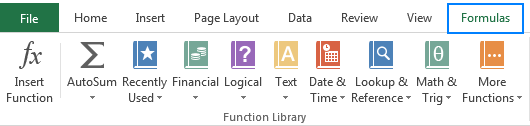There exist 400+ functions in Excel, and the number is growing by version to version. Of course, it's next to impossible to memorize all of them, and you actually don't need to. The Function Wizard will help you find the function best suited for a particular task, while the Excel Formula Intellisense will prompt the function's syntax and arguments as soon as you type the function's name preceded by an equal sign in a cell: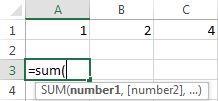Clicking the function's name will turn it into a blue hyperlink, which will open the Help topic for that function.

Tip. You don't necessarily have to type a function name in all caps, Microsoft Excel will automatically capitalize it once you finish typing the formula and press the Enter key to complete it.

## 10 Excel basic functions you should definitely know

What follows below is a list of 10 simple yet really helpful functions that are a necessary skill for everyone who wishes to turn from an Excel novice to an Excel professional.

### SUM

The first Excel function you should be familiar with is the one that performs the basic arithmetic operation of addition:

SUM(number1, [number2], …)

In the syntax of all Excel functions, an argument enclosed in [square brackets] is optional, other arguments are required. Meaning, your Sum formula should include at least 1 number, reference to a cell or a range of cells. For example:

`=SUM(B2:B6)` - adds up values in cells B2 through B6.

`=SUM(B2, B6)` - adds up values in cells B2 and B6.

If necessary, you can perform other calculations within a single formula, for example, add up values in cells B2 through B6, and then divide the sum by 5:

`=SUM(B2:B6)/5`

To sum with conditions, use the SUMIF function: in the 1st argument, you enter the range of cells to be tested against the criteria (A2:A6), in the 2nd argument - the criteria itself (D2), and in the last argument - the cells to sum (B2:B6):

`=SUMIF(A2:A6, D2, B2:B6)`

In your Excel worksheets, the formulas may look something similar to this: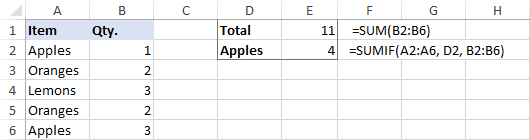Tip. The fastest way to sum a column or row of numbers is to select a cell next to the numbers you want to sum (the cell immediately below the last value in the column or to the right of the last number in the row), and click the AutoSum button on the Home tab, in the Formats group. Excel will insert a SUM formula for you automatically.

### AVERAGE

The Excel AVERAGE function does exactly what its name suggests, i.e. finds an average, or arithmetic mean, of numbers. Its syntax is similar to SUM's:

AVERAGE(number1, [number2], …)

Having a closer look at the formula from the previous section (`=SUM(B2:B6)/5`), what does it actually do? Sums values in cells B2 through B6, and then divides the result by 5. And what do you call adding up a group of numbers and then dividing the sum by the count of those numbers? Yep, an average!

The Excel AVERAGE function performs these calculations behind the scenes. So, instead of dividing sum by count, you can simply put this formula in a cell:

`=AVERAGE(B2:B6)`

To average cells based on condition, use the following AVERAGEIF formula, where A2:A6 is the criteria range, D3 is he criteria, and B2:B6 are the cells to average:

`=AVERAGEIF(A2:A6, D3, B2:B6)`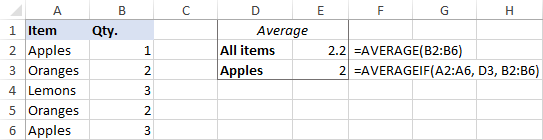### MAX & MIN

The MAX and MIN formulas in Excel get the largest and smallest value in a set of numbers, respectively. For our sample data set, the formulas will be as simple as:

`=MAX(B2:B6)`

`=MIN(B2:B6)`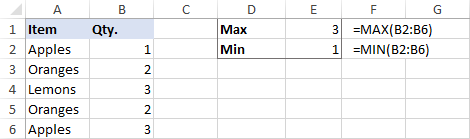### COUNT & COUNTA

If you are curious to know how many cells in a given range contain numeric values (numbers or dates), don't waste your time counting them by hand. The Excel COUNT function will bring you the count in a heartbeat:

COUNT(value1, [value2], …)

While the COUNT function deals only with those cells that contain numbers, the COUNTA function counts all cells that are not blank, whether they contain numbers, dates, times, text, logical values of TRUE and FALSE, errors or empty text strings (""):

COUNTA (value1, [value2], …)

For example, to find out how many cells in column B contain numbers, use this formula:

`=COUNT(B:B)`

To count all non-empty cells in column B, go with this one:

`=COUNTA(B:B)`

In both formulas, you use the so-called "whole column reference" (B:B) that refers to all the cells within column B.

The following screenshot shows the difference: while COUNT processes only numbers, COUNTA outputs the total number of non-blank cells in column B, including the the text value in the column header.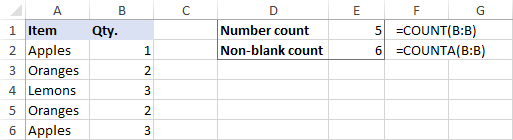### IF

Judging by the number of IF-related comments on our blog, it's the most popular function in Excel. In simple terms, you use an IF formula to ask Excel to test a certain condition and return one value or perform one calculation if the condition is met, and another value or calculation if the condition is not met:

IF(logical_test, [value_if_true], [value_if_false])

For example, the following IF statement checks if the order is completed (i.e. there is a value in column C) or not. To test if a cell is not blank, you use the "not equal to" operator ( <>) in combination with an empty string (""). As the result, if cell C2 is not empty, the formula returns "Yes", otherwise "No":

`=IF(C2<>"", "Yes", "No")`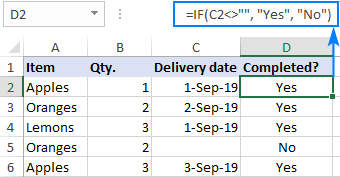### TRIM

If your obviously correct Excel formulas return just a bunch of errors, one of the first things to check is extra spaces in the referenced cells (You may be surprised to know how many leading, trailing and in-between spaces lurk unnoticed in your sheets just until something goes wrong!).

There are several ways to remove unwanted spaces in Excel, with the TRIM function being the easiest one:

TRIM(text)

For example, to trim extra spaces in column A, enter the following formula in cell A1, and then copy it down the column:

`=TRIM(A1)`

It will eliminate all extra spaces in cells but a single space character between words: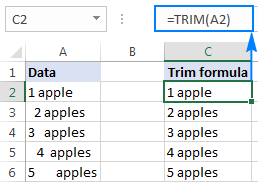### LEN

Whenever you want to know the number of characters in a certain cell, LEN is the function to use:

LEN(text)

Wish to find out how many characters are in cell A2? Just type the below formula into another cell:

`=LEN(A2)`

Please keep in mind that the Excel LEN function counts absolutely all characters including spaces: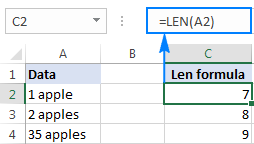Want to get the total count of characters in a range or cells or count only specific characters? Please check out the following resources.

### AND & OR

These are the two most popular logical functions to check multiple criteria. The difference is how they do this:

• AND returns TRUE if all conditions are met, FALSE otherwise.
• OR returns TRUE if any condition is met, FALSE otherwise.

While rarely used on their own, these functions come in very handy as part of bigger formulas.

For example, to check the test results in columns B and C and return "Pass" if both are greater than 60, "Fail" otherwise, use the following IF formula with an embedded AND statement:

`=IF(AND(B2>60, B2>60), "Pass", "Fail")`

If it's sufficient to have just one test score greater than 60 (either test 1 or test 2), embed the OR statement:

`=IF(OR(B2>60, B2>60), "Pass", "Fail")`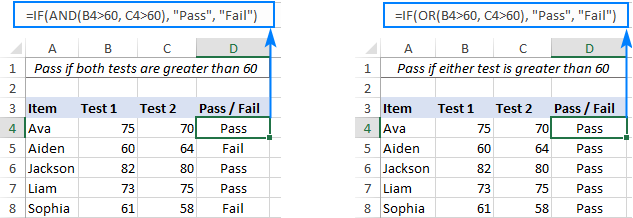### CONCATENATE

In case you want to take values from two or more cells and combine them into one cell, use the concatenate operator (&) or the CONCATENATE function:

CONCATENATE(text1, [text2], …)

For example, to combine the values from cells A2 and B2, just enter the following formula in a different cell:

`=CONCATENATE(A2, B2)`

To separate the combined values with a space, type the space character (" ") in the arguments list:

`=CONCATENATE(A2, " ", B2)`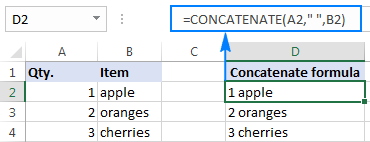### TODAY & NOW

To see the current date and time whenever you open your worksheet without having to manually update it on a daily basis, use either:

`=TODAY()` to insert the today's date in a cell.

`=NOW()` to insert the current date and time in a cell.

The beauty of these functions is that they don't require any arguments at all, you type the formulas exactly as written above.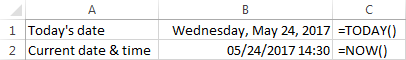## Best practices for writing Excel formulas

Now that you are familiar with the basic Excel formulas, these tips will give you some guidance on how to use them most effectively and avoid common formula errors.

### Do not enclose numbers in double quotes

Any text included in your Excel formulas should be enclosed in "quotation marks". However, you should never do that to numbers, unless you want Excel to treat them as text values.

For example, to check the value in cell B2 and return 1 for "Passed", 0 otherwise, you put the following formula, say, in C2:

`=IF(B2="pass", 1, 0)`

Copy the formula down to other cells and you will have a column of 1's and 0's that can be calculated without a hitch.

Now, see what happens if you double quote the numbers:

`=IF(B2="pass", "1", "0")`

At first sight, the output is normal - the same column of 1's and 0's. Upon a closer look, however, you will notice that the resulting values are left-aligned in cells by default, meaning those are numeric strings, not numbers! If later on someone will try to calculate those 1's and 0's, they might end up pulling their hair out trying to figure out why a 100% correct Sum or Count formula returns nothing but zero.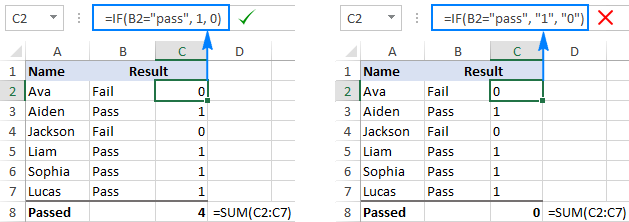### Don't format numbers in Excel formulas

Please remember this simple rule: numbers supplied to your Excel formulas should be entered without any formatting like decimal separator or dollar sign. In North America and some other countries, comma is the default argument separator, and the dollar sign (\$) is used to make absolute cell references. Using those characters in numbers may just drive your Excel crazy :) So, instead of typing \$2,000, simply type 2000, and then format the output value to your liking by setting up a custom Excel number format.

### Match all opening and closing parentheses

When crating a complex Excel formula with one or more nested functions, you will have to use more than one set of parentheses to define the order of calculations. In such formulas, be sure to pair the parentheses properly so that there is a closing parenthesis for every opening parenthesis. To make the job easier for you, Excel shades parenthesis pairs in different colors when you enter or edit a formula.

### Copy the same formula to other cells instead of re-typing it

Once you have typed a formula into a cell, there is no need to re-type it over and over again. Simply copy the formula to adjacent cells by dragging the fill handle (a small square at the lower right-hand corner of the cell). To copy the formula to the whole column, position the mouse pointer to the fill handle and double-click the plus sign.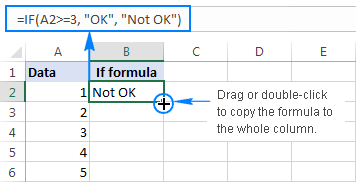Note. After copying the formula, make sure that all cell references are correct. Cell references may change depending on whether they are absolute (do not change) or relative (change).

For the detailed step-by-step instructions, please see How to copy formulas in Excel.

### How to delete formula, but keep calculated value

When you remove a formula by pressing the Delete key, a calculated value is also deleted. However, you can delete only the formula and keep the resulting value in the cell. Here's how:

• Select all cells with your formulas.
• Press Ctrl + C to copy the selected cells.
• Right-click the selection, and then click Paste Values > Values to paste the calculated values back to the selected cells. Or, press the Paste Special shortcut: Shift+F10 and then V.

For the detailed steps with screenshots, please see How to replace formulas with their values in Excel.

### Make sure Calculation Options are set to Automatic

If all of a sudden your Excel formulas have stopped recalculating automatically, most likely the Calculation Options somehow switched to Manual. To fix this, go to the Formulas tab > Calculation group, click the Calculation Options button, and select Automatic.

If this does not help, check out these troubleshooting steps: Excel formulas not working: fixes & solutions.

This is how you make and manage basic formulas in Excel. I how you will find this information helpful. Anyway, I thank you for reading and hope to see you on our blog next week.